RUSSIAN JOURNAL OF EARTH SCIENCES VOL. 11, ES2003, doi:10.2205/2009ES000338, 2009

## On mathematic formalization of similarity of records of electrical and seismic signals

M. D. Kovalenko
Institute of Physics of the Earth RAS, Moscow, Russia

A. D. Gvishiani, Sh. R. Bogoutdinov
Geophysical Center RAS, Moscow, Russia

P. Bernard
Institute of Physics of the Earth, France

J. Zlotnicki
Laboratory of Volcanology of the University of Clermont-Ferrand, France

### Extended abstract

A possible way of analysis of records of seismic and electrical signals is an application of the classical interpolation theory [1-3], and also well-developed methods of the theory of information transmission [4,5].

The authors had at their disposal seismic and electrical records. They represented tables of figures – signals, recorded by corresponding devices at discrete moments of time. At that, for each record of a seismic signal (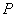wave) there were two records of an electric signal (a change of electrical resistivity of environment): one in orientation N-S, the other in orientation E-W.

To pass from a discrete set of tabular data to its analytical representation the Lagrange interpolation procedure was used [1,2]. This procedure to some extent amplifies the missing data, allowing to restore a continuous real-time signal according to an available discrete record to any desired degree of accuracy. It should be taken into account that any accompanying noise, related to the signal, would be also recorded. Thus, prior to signals' analysis, it would be desirable to remove any noise. Below S(x) – is a continuous seismic signal, and E(x) – a continuous electrical signal. According to  it can be recorded as(1)

Here Si , Ei – tabular values of measured seismic and electrical signals,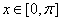– a current coordinate (recording time). The number records in interval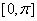, is equal to N + 1. Supposing that out of the interval all  Si , Ei equal to zero, then series (1) can be replaced by final sums with summation of series from i = 0 to i = N (assuming that electrical and seismic signals were recorded simultaneously, with a uniform pitch).

According to , an analytical dependence can be constructed, expressing electric signal through a seismic one. A corresponding formula (transfer function ) can be represented in the following way(2)

The theory of Lagrange interpolation [1, 5] proves that function E(x) can be reconstructed by function S(x) with the help of transfer function (2) to any desired degree of accuracy in the following way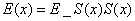(3)

Formula (3) has one obvious shortcoming – it is rather complicated. It can be simplified, obtaining the following expression, connecting electrical and seismic signals.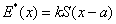(4)

Parameters a and k could be chosen in such a way that function E*(x) will be very closely approximated to function E(x). There are different approaches to defining these parameters. For example, in the present work parameter a was defined (graphically) according to the condition of a maximum of the correlation coefficient between initial electrical record and its approximate representation (4).

The fact that passing of wave P entails a drop in rock electrical resistivity is well known  and can be explained from a physical point of view. At the moment of passing of a tensile wave microfissures open and are filled with water (fluid), followed by a drop in electrical resistivity in proportion to a degree of opening of micropores. Parameter of shift a in formula (4) in fact represents the time, required for filling micropores with water.

References

1. Levin B. Y. (1956), Distribution of Finite Functions' Roots. M., Nauka, 682 p.

2. Akhiezer N. I. (1965), Lectures on the Theory of Approximations. M., GIFML, 407 p.

3. Kirillov A.A., Gvishiani A. (1982), Theorems and problems in functional analysis, New York-Heidelberg-Berlin, Springer-Verlag. Seria "Problem books in mathematics", 347 p.

4. Gvishiani A., Dubois J. (2002), Artificial Intelligence and Dynamic Systems for Geophysical Applications. Springer-Verlag, Paris, 350 p.

5. Yakovlev Y. I., Khurgin V. P. (1971), Finite Functions in Physics and Technology. M, GIFML, 408 p.

6. G. A. Sobolev, A. V. Ponomarev. Physics of Earthquakes and Precursors. M., Nauka. 2003. 270 p.

Received 13 October 2009; accepted 28 October 2009; published 30 November 2009.

Keywords: seismic and electrical signals, analytical dependencies, Lagrange interpolationCitation: Kovalenko M. D., A. D. Gvishiani, Sh. R. Bogoutdinov, P. Bernard, J. Zlotnicki (2009), On mathematic formalization of similarity of records of electrical and seismic signals, Russ. J. Earth Sci., 11, ES2003, doi:10.2205/2009ES000338.

Copyright 2009 by the Russian Journal of Earth Sciences